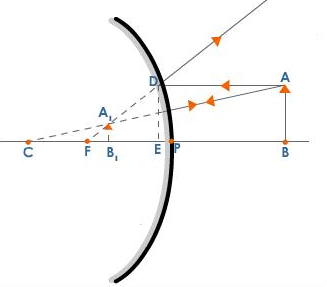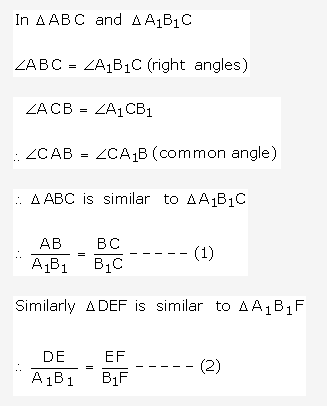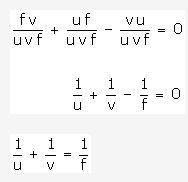# Derive mirror formula for a convex mirror

derive mirror formula for a convex mirror.

Let AB be an object placed on the principal axis of a convex mirror of focal length f. u is the distance between the object and the mirror and v is the distance between the image and the mirror.But DE = AB and when the aperture is very small EF = PF.Equation (2) becomesFrom equations (1) and (3) we get[PF = f, PB1 = v, PB = u, PC = 2f]Dividing both sides of the equation (4) by uvf we get